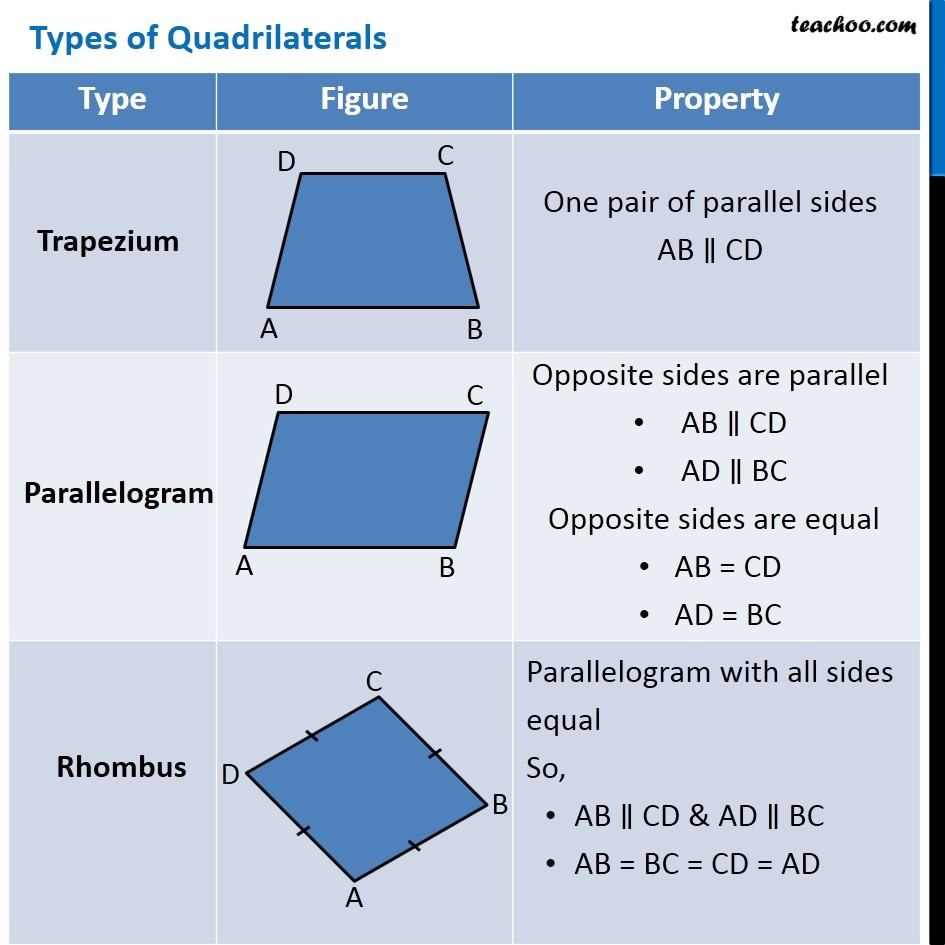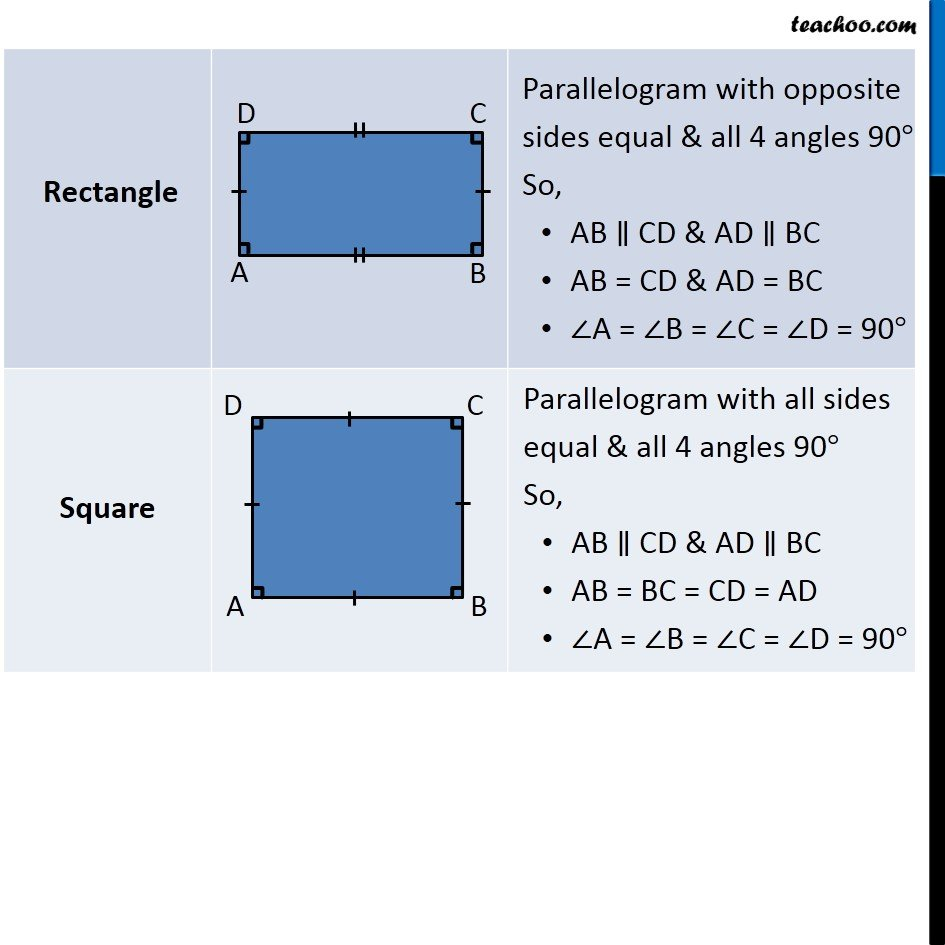## Trapezium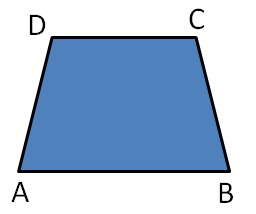One pair of parallel sides

AB ∥ CD

## Parallelogram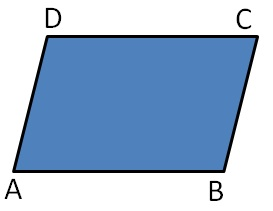Opposite sides are parallel

• AB ∥ CD
• AD ∥ BC

Opposite sides are equal

• AB = CD
• AD = BC

## Rhombus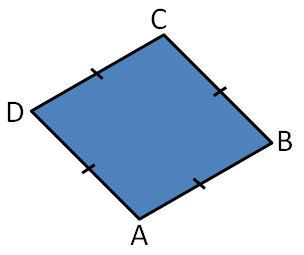Parallelogram with all sides equal

So,

• AB ∥ CD & AD ∥ BC
• AB = BC = CD = AD

## Rectangle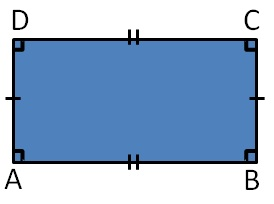Parallelogram with opposite sides equal & all 4 angles 90°

So,

• AB ∥ CD & AD ∥ BC
• AB = CD & AD = BC
• ∠A = ∠B = ∠C = ∠D = 90°

## Square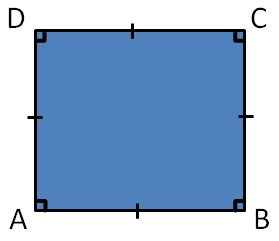Parallelogram with all sides equal & all 4 angles 90°

So,

• AB ∥ CD & AD ∥ BC
• AB = BC = CD = AD
• ∠A = ∠B = ∠C = ∠D = 90°
1. Chapter 8 Class 9 Quadrilaterals
2. Concept wise

Transcript

One pair of parallel sides AB ∥ CD Two pairs of parallel lines AB ∥ CD AD ∥ BC Opposite two sides are equal AB = CD AD = BC Parallelogram with all sides equal & all 4 angles 90° So, AB ∥ CD & AD ∥ BC AB = BC = CD = AD ∠A = ∠B = ∠C = ∠D = 90° Parallelogram with all sides equal & all 4 angles 90° So, AB ∥ CD & AD ∥ BC AB = CD = AD = BC ∠A = ∠B = ∠C = ∠D = 90° Parallelogram with all sides equal & all 4 angles 90° So, AB ∥ CD & AD ∥ BC AB = BC = CD = AD ∠A = ∠B = ∠C = ∠D = 90°

About the AuthorDavneet Singh
Davneet Singh is a graduate from Indian Institute of Technology, Kanpur. He has been teaching from the past 10 years. He provides courses for Maths and Science at Teachoo.